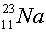Notation Nuclei are composed of neutrons and protons. A nucleus could specified by its number N of neutrons and its number of protons Z. A third symbol A refers to the netnumber of nucleons, A = N + Z. A nucleon is either a proton or a neutron. Elements are given names (e.g. carbon, lithium, potassium) according to their charge. Thus all carbon nuclei have Z = 6, and all gold nuclei have Z = 79. The word isotope refers to a specific combination of N and Z. If two carbon nuclei have different number of neutrons, one would say they are different isotopes of carbon. We demonstrate the notation for an isotope by showing the symbol for a sodium (all sodium has Z = 11) nucleus with 12 neutrons.which is the same as 23Na  because the 11 is redundant with the sodium symbol Since the upper left number refers to A, one must subtract Z from A to find the number of neutrons, N. To obtain the charge of a nucleus, one can look at a periodic table. Since electrons are bound by the Coulomb force which is not affected by the number of neutrons, a periodic table does not usually mention different isotopes of the same element. Nuclear physicists use a table of the nuclides, rather than a periodic table to classify nuclei.